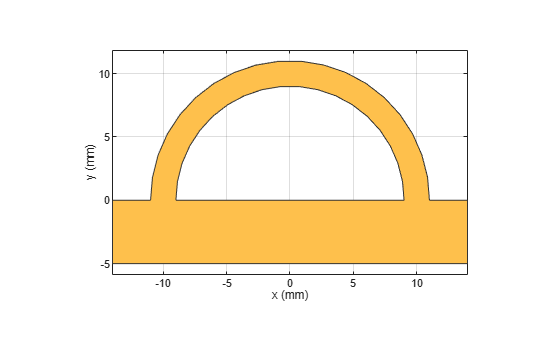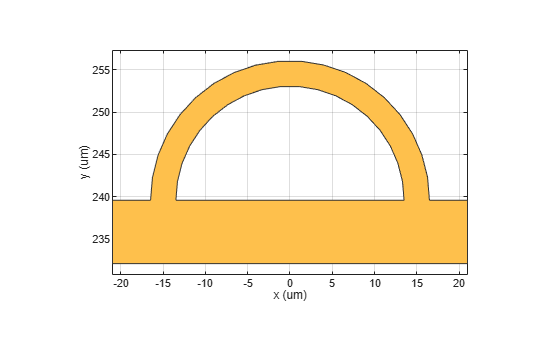scale

Change size of RF PCB shape by fixed amount

Description

example

c = scale(shape,scaleFactor) resizes the shape by a scaling factor.

c = scale(shape,scaleFactor,RefPoint) scales the shape by a constant factor with respect to the reference point. The reference point is ignored if the shape is symmetrical and scale is performed based on centroid. The reference point is considered if the shape is unsymmetrical and scale is performed based on specified reference point.

Examples

collapse all

Create a shape consisting of a curve and a right angle U-bend.

shape1 = curve;
shape2 = ubendRightAngle(Length=[5 18 5]*1e-3,ReferencePoint=[0 -5]*1e-3);
shapeSum = shape1+shape2;

Display the shape.

show(shapeSum)Specify a scale factor, then resize the shape. Display the result.

s = 1.5*1e-3;
shapeTrans = scale(shapeSum,s);
show(shapeTrans)Input Arguments

collapse all

RF PCB shape created using custom elements and shape objects of RF PCB Toolbox™, specified as an object.

Example: shape = bendCurved; specifies the shape as a bendCurved object.

Scaling factor to change shape size, specified as a scalar.

Data Types: double